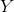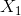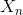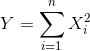GRE Subject Test: Math : Chi-Squared Distribution

Example Questions

Example Question #1 : Chi Squared Distributionfollows a chi-squared distribution withdegrees of freedom andthroughare independent standard normal variables.

If, what is?Use the fact that the sum ofsquared standard and independent normal variables follows a chi-squared distribution withdegrees of freedom.